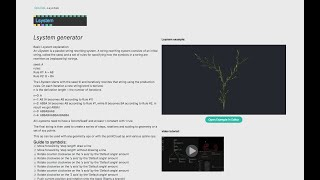beta
cables is under heavy development.
There might be one or another bug, please let us know about it!

# Lsystem_v2 Op

`Ops.Systems.Lsystem_v2`Collections:generator
Namespace: Ops.Systems

Op author: andro

### Lsystem generator

summary (oneliner)

Basic Lsystem explanation:
An LSystem is a parallel string rewriting system. A string rewriting system consists of an initial string, called the seed, and a set of rules for specifying how the symbols in a string are rewritten as (replaced by) strings.

seed: A
rules:
Rule #1: A = AB
Rule #2: B = BA

The LSystem starts with the seed ‘A’ and iteratively rewrites that string using the production rules. On each iteration a new string/word is derived.
n is the derivation length = the number of iterations

n=0: A
n=1: AB (A becomes AB according to Rule #1)
n=2: ABBA (A becomes AB according to Rule #1, while B becomes BA according to Rule #2. In result we get ABBA)
n=3: ABBABAAB
n=4: ABBABAABBAABABBA

All Lsystems need to have a 'Axiom/Seed' and at least 1 constant with 1 rule

The final string is then used to create a series of steps, rotations and scaling to geometry or a set of xyz points.

This op can be used with any geometry ops or with the pointCloud op and various spline ops.

#### Guide to symbols:

`F` Move forward by 'step length' draw a line
`f` Move forward by 'step length' without drawing a line
`x` Rotate counter clockwise on the 'x axis' by the 'Default angle' amount
`X` Rotate clockwise on the 'y axis' by the 'Default angle' amount
`y` Rotate counter clockwise on the 'y axis' by the 'Default angle' amount
`Y` Rotate clockwise on the 'y axis' by the 'Default angle' amount
`z` Rotate counter clockwise on the 'z axis' by the 'Default angle' amount
`Z` Rotate clockwise on the 'Z axis' by the 'Default angle' amount
`[` Push current position and rotation onto the stack (Starts a branch)
`]` Pop current position and rotation from the stack (Closes a branch)
`>` Multiply the line length by the 'Step scale Multiplier'
`<` Divide the line length by the 'Step scale Multiplier'

Each rotation can also be assigned a different value than the 'Default Angle' by typing in the axis and then a number.

`x34.5` Will rotate -34.5 degrees on the x axis

User defined angles and default angles can be mixed together like this
`FFx34.5FyzFx20.2`

To create 2 branches:
`FF[xFFyzF]F[yxFFyzF]`

To nest one branch inside another:
`FF [xFF [YzFF] fF ]`

All branches must be closed to generate a valid string.
[FFxF] / this will work
[FFXF / this won't due to the missing close branch symbol

Check http://paulbourke.net/fractals/lsys/ for Rule sets and examples

doc

issues

example patch id

caniuse query

collections

related ops

## Inputs

Trigger (Trigger)

Trigger the op

Axiom / seed (String)

The start of the generated string

Constant 1 (String)

If this is encountered in the string Rule 1 is applied

Rule 1 (String)

Constant 2 (String)

If this is encountered in the string Rule 2 is applied

Rule 2 (String)

Constant 3 (String)

If this is encountered in the string Rule 3 is applied

Rule 3 (String)

Constant 4 (String)

If this is encountered in the string Rule 4 is applied

Rule 4 (String)

Iterations (integer /Number)

Iteration amount, be careful with high numbers, the generated string is highly dependent on the Constants and Rules.

Step length (Number)

How far the current point moves when "F" or "f" is used

Step scale multiplier (Number)

Used to multiply or divide the current 'step length' whenever ">" or "<" is used

Default angle (Number)

The rotation amount that is used when a rotation amount isn't defined

Rotation multiplier (Number)

Multiplies all default angles by this amount

random seed (Number)

Seed used for "Random Strength" amount

random strength (Number)

Amount of random offset applied to position and rotation of every point

## Outputs

Out trigger geometry (Trigger)

Used to trigger geometry

Line/point trigger (Trigger)

Used to trigger pointCloudArray or SimpleSpline

Points out (Array)

An array containing all the xyz data for every point

Max Size (Number)

The largest distance from the starting point, can be used to make sure that the generated Lsystem is always within a bounding box. Check example file to see how this works.

Final generated string (String)

The final string used to create the Lsystem

Caught a mistake or want to contribute to the documentation?

### Lsystem cyber 01

Youtube Video Tutorial## Patches using Lsystem_v2

• Examples
• Public

#### Changelog

created op
2019-10-02 - andro

Ops.Exp.Lsystem_v2 renamed to Ops.Systems.Lsystem_v2
2019-10-15 - pandur